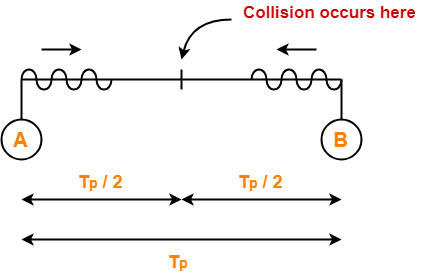2,099 views

Consider two nodes A and B on the same ethernet segment, and suppose the propagation delay between two nodes is 225 bit times. Suppose at time both nodes A and B begin to transmit a frame. Assume both nodes transmit a 50 bit jam signal after detection a collision.

For 107 bits per set ethernet , find the time at which both nodes A and B sense an idle channel(in microsec) ?

### 1 comment

50 micro sec.??

BW = 10^7 bits/sec

PT {propogation time} =22.5 micro sec

In worst case, collision occurred at PT and Detected by transmitting node after 2PT = 2*22.5 =45 microsec

after detecting collision, 50bit jamming signal is transmitted that will take 5 microsec

time at which node A and B finds channel idle is 45+5= 50 microsec

@priyam Yes  u r right

both nodes are transmitting data at same time then collision detection will take Tp  only not 2*Tp.

right??

this is the correct explanation https://gateoverflow.in/147526/ethernet

Station A and B not try to transmit first bit of JAM signal.

These stations  will detect Channel free after Jam signal is transmitted in whole channel i.e. 2* (propagation delay) + transmission time of Jam signal

Tp=$\frac{225 bits}{10^{7} bits/sec}$ = 22.5 micro seconds

Transmission time of Jam signal = $\frac{50 bits}{10^{7} bits/sec}$ = 5 micro seconds

so

the time at which both nodes A and B sense an idle channel = 2* 22.5 + 5 = 50 micro seconds

Since both the channels start transmitting at the same time, collision will occur when the signal is halfway so , collision occurs at 112.5 bit time and then it takes 112.5 bit time again for the collision information to reach respective nodes. Therefore, collision is detected at 225 bit time.

Bit time can be converted to secs as :  $\frac{225}{10^7} sec$ = 22.5 μs

Now they will transmit 50 bit jam signal. To transmit 50 bits we need $\frac{50}{10^7} sec$ = 5 μs

The jamming signal again takes 225 bit time to propagate to the other end. i.e 22.5 μs

Therefore total time after which the channel will be clear = 22.5 + 5 + 22.5 = 50 μs

by

Propagation delay (Tp)

= 225 bit times

= 225 bit / 10  6

= 22.5 x 10-6 sec

= 22.5 μsec

### At t = 0,

• Nodes A and B start transmitting their frame.
• Since both, the stations start simultaneously, so collision occurs in the midway.
• Time after which collision occurs = Half of the propagation delay.
• So, time after which collision occurs = 22.5 μsec / 2 = 11.25 μsec.### At t = 11.25 μsec,

• After the collision occurs at t = 11.25 μsec, collided signals start traveling back.
• Collided signals reach the respective nodes after time = Half of propagation delay
• Collided signals reach the respective nodes after time = 22.5 μsec / 2 = 11.25 μsec.
• Thus, at t = 22.5 μsec, collided signals reach the respective nodes.

### At t = 22.5 μsec,

• As soon as nodes discover the collision, they immediately release the jamming signal.
• Time taken to finish transmitting the jam signal = 50 bit time = 50 bits/ 10 Mbps = 5 μsec.

Thus,

Time at which the jamming signal is completely transmitted

= 22.5 μsec + 5 μsec

= 27.5 μsec

by